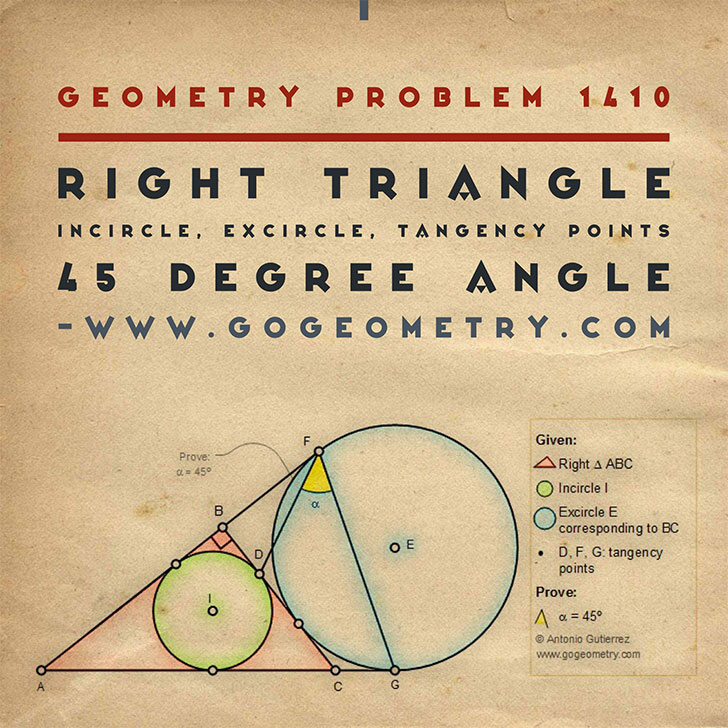# Geometry Problem 1410: Right Triangle, Incircle, Excircle, Tangency Points, 45 Degree Angle

### Proposition

The figure below shows a right triangle ABC (angle B = 90 degree) with the incircle I tangent to BC at D. The excircle E corresponding to BC is tangent to AB and AC extended at F and G, respectively. Prove that the measure of the angle DBG is 45 degree.### Poster of Problem 1410: Sketching, iPad, Typography, Art